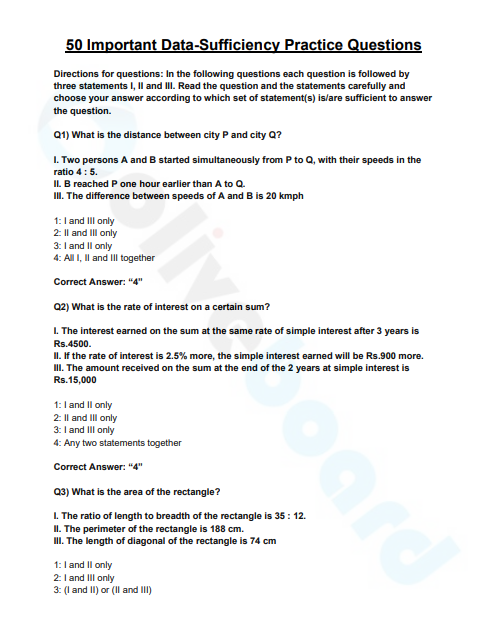Important Data Sufficiency Questions : Data Sufficiency is one of the important topics that demand practice. In data sufficiency, usually, a question is followed by two or three statements. You need to determine whether any of the statements individually or together are required to find the answer. In this blog, we have brought you a free e-book on 50 Important Data Sufficiency Questions.

Download the free ebook on Data Sufficiency questions. Want To Attempt A Free Mock Test? Register Here & Attempt A Free Full-Length Mock Test

Click on the link provided below and get the complete free PDF.Here is a complete step by step procedure to download the PPB Questions PDF.

Step 2: Register/Login to the Free E-Books Page of Oliveboard (It is 100% free, You just enter your valid email ID and a password to be able to download the Data Sufficiency PDF.

Step 3: After Logging in, you will be able to download the free e-book by clicking on “click here” as shown in the snap below.

## Here’s A Sneek Peek Into E-book

Directions for questions: In the following questions each question is followed by three statements I, II, and III. Read the question and the statements carefully and choose your answer according to which set of the statement(s) is/are sufficient to answer the question.

Q1) What is the distance between city P and city Q?

I. Two persons A and B started simultaneously from P to Q, with their speeds in the
ratio 4 : 5.
II. B reached P one hour earlier than A to Q.
III. The difference between speeds of A and B is 20 kmph

1: I and III only
2: II and III only
3: I and II only
4: All I, II and III together

Q2) What is the rate of interest on a certain sum?

I. The interest earned on the sum at the same rate of simple interest after 3 years is Rs.4500.
II. If the rate of interest is 2.5% more, the simple interest earned will be Rs.900 more.
III. The amount received on the sum at the end of the 2 years at simple interest is Rs.15,000.

1: I and II only
2: II and III only
3: I and III only
4: Any two statements together

Want to attempt a free mock test? Take A Free Mock Test Here

Q3) What is the area of the rectangle?

I. The ratio of length to breadth of the rectangle is 35 : 12.
II. The perimeter of the rectangle is 188 cm.
III. The length of diagonal of the rectangle is 74 cm

1: I and II only
2: I and III only
3: (I and II) or (II and III)
4: Any two of the three

Q4) What is the present age of Rakesh?

I. The ratio of the ages of Ramesh and Rakesh, three years ago, was 4 : 5.
II. The ratio of the ages of Rajesh and Rakesh, after two years, will be 4 : 5.
III. Two years ago the ratio of the ages of Rajesh and Ramesh was 12 : 13.

1: I and II only
2: II and III only
3: I and III only
4: All I, II and III

## What’s there in this E-book?Want to attempt a free mock test? Take A Free Mock Test Here

## Grab Free eBooks On Our Platform

You can download 200+ ebooks important for SSC, Banking, Railway, Insurance, and other government exams here.Oliveboard Bolt series E-books: